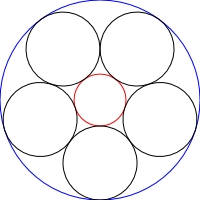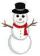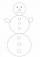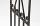# Annulus

Two concentric circles with radii 1 and 9 surround the annular circle. This ring is inscribed with n circles that do not overlap. Determine the highest possible value of n.

Correct result:

n =  3

#### Solution:We would be pleased if you find an error in the word problem, spelling mistakes, or inaccuracies and send it to us. Thank you!Tips to related online calculators

#### You need to know the following knowledge to solve this word math problem:

We encourage you to watch this tutorial video on this math problem:

## Next similar math problems:

• DodecagonCalculate the size of the smaller of the angles determined by lines A1 A4 and A2 A10 in the regular dodecagon A1A2A3. .. A12. Express the result in degrees.
• Top of the towerThe top of the tower has the shape of a regular hexagonal pyramid. The base edge has a length of 1.2 m, the pyramid height is 1.6 m. How many square meters of sheet metal is needed to cover the top of the tower if 15% extra sheet metal is needed for joint
• Sphere in coneA sphere is inscribed in the cone (the intersection of their boundaries consists of a circle and one point). The ratio of the surface of the ball and the contents of the base is 4: 3. A plane passing through the axis of a cone cuts the cone in an isoscele
• Circumferential angleVertices of the triangle ΔABC lies on circle and divided it into arcs in the ratio 2:2:9. Determine the size of the angles of the triangle ΔABC.
• Candy - MOGretel deploys to the vertex of a regular octagon different numbers from one to eight candy. Peter can then choose which three piles of candy give Gretel others retain. The only requirement is that the three piles lie at the vertices of an isosceles trian
• A cylinderA cylinder 108 cm high has a circumference of 24 cm. A string makes exactly 6 complete turns around the cylinder while its two ends touch the cylinder's top and bottom. (forming a spiral around the cylinder). How long is the string in cm?
• Snowman 2On the medal, which has the shape of a circle with a diameter 18 cm is sketched snowman so that the following requirements are met: 1. snowman is composed of three circles, 2. space over snowman is the same as under it, 3. diameters of all circles express
• RightDetermine angles of the right triangle with the hypotenuse c and legs a, b, if: ?
• SnowmanIn a circle with a diameter 50 cm are drawn 3 circles /as a snowman/ where: its diameters are integers, each larger circle diameter is 3 cm larger than the diameter of the previous circle. Determine snowman height if we wish highest snowman.
• HexagonThere is regular hexagon ABCDEF. If area of the triangle ABC is 22, what is area of the hexagon ABCDEF? I do not know how to solve it simply....
• MastMast has 13 m long shadow on a slope rising from the mast foot in the direction of the shadow angle at angle 13.3°. Determine the height of the mast, if the sun above the horizon is at angle 45°12'.
• Eq triangle minus arcsIn an equilateral triangle with a 2cm side, the arcs of three circles are drawn from the centers at the vertices and radii 1cm. Calculate the content of the shaded part - a formation that makes up the difference between the triangle area and circular cutsCalculate the content of a regular 15-sides polygon inscribed in a circle with radius r = 4. Express the result to two decimal places.To a circle is inscribed triangle so that the it's vertexes divide circle into 3 arcs. The length of the arcs are in the ratio 2:3:7. Determine the interior angles of a triangle.The base of the pool is a circle with a radius r = 10 m, excluding a circular segment that determines the chord length 10 meters. The pool depth is h = 2m. How many hectoliters of water can fit into the pool?The room is 10 x 5 meters. You have the role of carpet width of 1 meter. Make rectangular cut of roll that piece of carpet will be longest possible and it fit into the room. How long is a piece of carpet? Note .: carpet will not be parallel with the diagoScouts have a tent in the shape of a regular quadrilateral pyramid with a side of the base 4 m and a height of 3 m. Determine the radius r (and height h) of the container so that they can hide the largest possible treasure.